×
Get Full Access to ECU - FINA 3724 - Class Notes - Week 3
Get Full Access to ECU - FINA 3724 - Class Notes - Week 3

×

ECU / Finance / FINA 3724 / the quickfixcompany just paid a dividend of \$1.25 and analysts expect

# the quickfixcompany just paid a dividend of \$1.25 and analysts expect Description

##### Description: This includes all of chapter 7 material and the chapter 7 additional problems with solutions.
13 Pages 408 Views 0 Unlocks
Reviews

## How much should he offer to buy the stock at?## If Joe wants to earn an annual rate of return of 12% on this investment, how much should he offer to buy the stock at?## In order to earn 12% on this investment, how much should Agnes pay for this stock?FINA 3724 Chapter 7 Notes: Stocks and Stock Valuation 7.1 Characteristics of Common Stock ∙ Major financing vehicle for corporations  ∙ Provides holders with an opportunity to share in the future cash flows  of thIf you want to learn more check out Homeostasis is maintained in a 3 part system. What are they?
If you want to learn more check out What is an example of a complex number.
If you want to learn more check out What are the steps in making extemporaneous speech?
If you want to learn more check out Why do we pitch a campaign proposal to the client?
We also discuss several other topics like What is a mathematical representation of an experiment that produces real numbers?
If you want to learn more check out What is meant by coupon payment?
e issuer.  ∙ Holders have ownership in the company. ∙ Unlike bonds, no maturity date and variable periodic income 7.1 (A) Ownership ∙ Share in the residual profits of the company. ∙ Claim to all its assets and cash flow once the creditors, employees,  suppliers, and taxes are paid off.  ∙ Voting rights  o participate in the management of the company o elect the board of directors which selects the management team  that runs the company’s day-to-day operations. 7.1 (B) Claim on Assets and Cash Flow (Residual Claim) ∙ In case of liquidation: Shareholders have a claim on the residual assets  and cash flow of the company. (Known as “residual” rights) 7.1 (C) Vote (Voice in Management) ∙ Standard voting rights: Typically, one vote per share provided to  shareholders to vote in board elections and other key changes to the  charter and bylaws.  ∙ Can be altered by issuing several classes of stock. o Non-voting stock, which is usually for a temporary period of time o Super voting rights, which provide the holders with multiple  votes per share, increasing their influence and control over the  company. 7.1 (D) No Maturity Date ∙ Considered to be permanent financing ∙ Infinite life, i.e. no maturity date ∙ No promised date when investment is returned. 7.1 (E) Dividends and Their Tax Effect ∙ Companies pay cash dividends periodically (usually every quarter) to  their shareholders out of net income.  ∙ Unlike coupon interest paid on bonds, dividends cannot be treated as a tax-deductible expense by the company.  ∙ For the recipient, however, dividends are considered to be taxable  income. 7.1 (F) Authorized, Issued, and Outstanding Shares ∙ Authorized shares: maximum number of shares that the company may  sell, as per charter.  ∙ Issued shares: the number of shares that has already been sold by  the company and are either currently available for public trading  (outstanding shares) or held by the company for future uses such as  rewarding employees (treasury stock) 7.1 (G) Treasury Stock ∙ Non-dividend paying, non-voting shares being held by the issuing firm  right from the time they were first issued ∙ Shares that have been later repurchased by the issuing firm in the  market. 7.1 (H) Preemptive Rights ∙ Privileges that allow current shareholders to buy a fixed percentage of  all future issues before they are offered to the general public. ∙ Enables current common stockholders to maintain their proportional  ownership in the company.  7.2 Stock Markets Stocks are traded in two types of markets: 1. Primary (First Sale) Market 2. Secondary (After-Sale) Market 7.2 (A) Primary Markets First issue market where using firm is involved. ∙ Initial public offering (IPO): first public equity issue; investment banker  becomes a partner and will use the talent/expertise of the bank’s  management to structure the sale and comply with all SEC regulations ∙ Prospectus: a document that provides potential buyers with  information about the company and the impending sale ∙ Due diligence: ensures disclosure of all relevant information prior to  the sale ∙ Firm commitment: the investment banker essentially buys the entire  stock issue from the company at one price and then sells the issue for  a higher price ∙ Best efforts: investment banker pledges to give his or her best in trying to sell the shares and will take a small percentage of the sale of each  stock; does not guarantee the firm a specific amount from the sale7.2 (B) Secondary Markets: How stocks trade ∙ Forum where common stock can be traded among investors  themselves. ∙ Provides liquidity and variety. ∙ In the United States, 3 well-known secondary stock markets: o NYSE: New York Stock Exchange o AMEX: American Stock Exchange o NASDAQ: National Association of Securities Dealers Automated  Quotation System  Specialist: maintain an orderly market for stock  Ask price: price at which he/she wants to sell  Bid price: price at which he/she is willing to buy  Bid-ask spread: difference between the asking price and  bidding price 7.2 (C) Bull Markets and Bear Markets ∙ Bull market: prolonged rising stock market, coined on the analogy that  a bull attacks with his horns from the bottom up. ∙ Bear market: prolonged declining market, based on the analogy that a  bear swipes with his paws from the top down. 7.3 Stock Valuation ∙ Value of a share of stock  the present value of its expected future  cash flow… o Cash dividends paid (if any).  o Future selling price of the stock. o The discount rate i.e. risk-appropriate rate of return to be earned  on the investment. ∙ No guaranteed cash flow information. ∙ No maturity date. ∙ Valuation is more of an “art” than a science. Table 7.1 Differences between Bonds and Stocks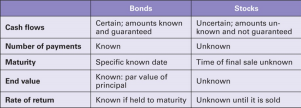7.3 Example 1: Stock price with known dividends and sale price. Agnes wants to purchase common stock of New Frontier Inc. and hold it for 3  years. The directors of the company just announced that they expect to pay  an annual cash dividend of \$4.00 per share for the next 5 years. Agnes believes that she will be able to sell the stock for \$40 at the end of three  years. In order to earn 12% on this investment, how much should Agnes pay  for this stock? Solution: Method 2 (Financial Calulator) Input:N I/Y PV PMT FV Key: 4 12 ? 4 40 Output: -37.57 4 Variations of a dividend pricing model have been use to value common  stock 1. The constant dividend model with an infinite horizon 2. The constant dividend model with a finite horizon 3. The constant growth dividend model with a finite horizon 4. The constant growth dividend model with an infinite horizon 7.3 (A) The Constant Dividend Model with an Infinite Horizon Assumes that the firm is paying the same dividend amount in perpetuity. i.e. Div1 = Div2 = Div3 = Div4 = Div5 = Div∞ For perpetuities: PV = PMT/r (where r the required rate and PMT is the cash flow.) Thus, for a stock that is expected to pay the same dividend forever: Price =  Dividend/Required rate of return Example: Quarterly Dividends Forever Let’s say that the Peak Growth Company is paying a quarterly dividend of  \$0.50 and has decided to pay the same amount forever. If Joe wants to earn  an annual rate of return of 12% on this investment, how much should he  offer to buy the stock at? Solution: Quarterly dividend = \$0.50 Quarterly rate of return = Annual rate/4= 12%/4 = 3% PV = Quarterly dividend/Quarterly rate of return Price = 0.50/.03 = \$16.67 7.3 (B) The Constant Dividend Model with a Finite Horizon ∙ Assumes that the stock is held for a finite period of time and then sold  to another investor.  ∙ Constant dividends received over the investment horizon. ∙ Price estimated as the sum of the present value of an annuity  (constant dividend) and that of a single sum (the selling price). ∙ Similar to a typical non-zero coupon, corporate bond.  ∙ Have to estimate the future selling price, since that is not a given  value, unlike the par value of a bond.Example: Constant dividends with finite holding period Let’s say that the Peak Growth Company is paying an annual dividend of  \$2.00 and has decided to pay the same amount forever.  Joe wants to earn an annual rate of return of 12% on this investment, and  plans to hold the stock for 5 years, with the expectation of selling it for \$20  at the end of 5 years. How much should he offer to buy the stock at? Solution: Annual dividend = \$2.00 = PMT Selling Price = \$20 = FV Annual rate of return = 12% PV = PV of dividend stream over 5 years + PV of Year 5 price Mode: P/Y= 1; C/Y=1 Input:N I/Y PV PMT FV Key: 5 12 ? 2 20 Output: -18.56 7.3 (C) The Constant Growth Dividend Model with an Infinite Horizon ∙ Known as the Gordon model (after its developer, Myron Gordon). ∙ Estimate is based on the discounted value of an infinite stream of  future dividends that grow at a constant rate, g.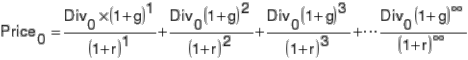∙ R = the required rate of return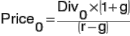∙ With some algebra, this can be simplified to…. ∙ And since Div0 * (1+g) = Div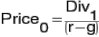1 :  ∙ Or more generally Pn = Divn+1/(r-g) Example: Constant growth rate, infinite horizon (with growth rate given) Let’s say that the Peak Growth Company just paid its shareholders an annual  dividend of \$2.00 and has announced that the dividends would grow at an  annual rate of 8% forever. If investors expect to earn an annual rate of  return of 12% on this investment how much would they offer to buy the stock for? Solution:Div0 = \$2.00; g=8%; r=12% Div1=Div0*(1+g)  Div1=\$2.00*(1.08)  Div1=\$2.16 P0 = Div1/(r-g)  \$2.16/(.12 - .08)  \$54  Price0 = \$54 ***Note: R &G must be in decimals Example: Constant growth rate, infinite horizon (with growth rate estimated  from past history) Let’s say that you are considering an investment in the common stock of  QuickFix Enterprises and are convinced that its last paid dividend of \$1.25  will grow at its historical average growth rate from here on. Using the past 10 years of dividend history and a required rate of return of 14%, calculate the  price of QuickFix’s common stock.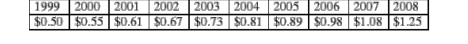Find Growth Rate and price of the stock (RROR = 14%) Solution: Compound growth rate “g” = (FV/PV)1/n -1 Where FV = \$1.25; PV = 0.50; n = 9 g = (1.25/0.50)1/9 – 1  10.72% Div1 = Div0(1+g)  \$1.25*(1.1072)  \$1.384 P0 = Div1/(r-g)  \$1.384/(.14-.1072) \$42.19 7.3 (D) The Constant Growth Dividend Model with a Finite Horizon Investor expects to hold a stock for a limited number of years, Company’s  dividends are growing at a constant rate. Following formula is used to value  the stock…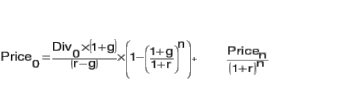Note: This formula would lead to the same price estimate as the Gordon  model, if it is assumed that the growth rate of dividends and the required  rate of return of the next owner, (after n years) remain the same. Example: Constant Growth, finite horizon. The QuickFix Company just paid a dividend of \$1.25 and analysts expect the  dividend to grow at its compound average growth rate of 10.72% forever.  If you plan on holding the stock for just 7 years, and you have an expected  rate of return of 14%, how much would you pay for the stock?  Assume that the next owner also expects to earn 14% on his or her  investment. We can solve this in 2 ways. 1. Method 1: Use the constant growth, finite horizon formula 2. Method 2: Use the Gordon Model since g is constant forever, and both  investors have the same required rates of returnSolution: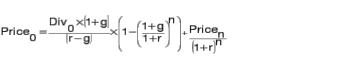Method 1:  Price in year 7 = Div8/(r-g) Div0 = \$1.25; g =10.72%; r=14%;  Div8 = D0(1+g)8 Div8  1.25*(1.1072)8 = 2.25844  P7=2.25844/(.14-.1072)  \$86.07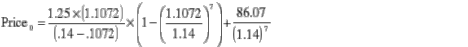 = \$42.195 *0.184829 + 34.40 = \$42.19 Method 2: Gordon Model P0 = D0(1+g)/(r-g) P0 = \$1.25*(1.1072)/(.14-.1072) P0 = \$42.19 7.3 (E) Non-Constant Growth Dividends ∙ The above 4 models work if a firm is either expected to pay a constant  dividend amount indefinitely, or is expected to have its dividends grow  at a constant rate for long periods of time.  ∙ For most firms, the dividend growth pattern tends to be variable,  making the valuation process complex.  ∙ However, if we can assume that at some point in the future, dividend  growth will become constant, we can use a combination of the Gordon  Model and present value equations to calculate the price of the stock. Example: Non-constant dividend pattern The Rapid Growth Company is expected to pay a  dividend of \$1.00 at the end of this year. Thereafter, the dividends are  expected to grow at the rate of 25% per year for 2 years, and then drop to  18% for 1 year, before settling at the industry average growth rate of 10%  indefinitely.  If you require a return of 16% to invest in a stock of this risk level, how much  would you be justified in paying for this stock? ∙ D1=\$1.00; g1=25%; n1=2; g2=18%; n2=1; gc=10%; r=16%  Solution: 1. Step 1. Find the annual non-constant growth dividends (Years 1-4 in  this case) using the appropriate growth rates. a. D4=\$1.56*(1.18) = \$1.84 2. Step 2. Find the first constant growth dividend (D5 in this case) and  use it to find the price of the stock once the dividend starts growing at  a constant rate using the Gordon modela. P4 = D5/(r-g) = D4(1+g)/(r-g) = \$1.84*(1.10)/(.16-.10) =  \$2.02/.06 = \$33.73 3. Step 3. Discount the annual dividends in Years 1-4 and the Price at the end of Year 4, back to Year 0 using the required rate of return as the  discount rate, and add them up to solve for the current price. (Draw a  timeline!) a. P0 = \$1.00/(1.16) + 1.25/(1.16)2+\$1.56/(1.16)3+\$1.84/(1.16)4+ \$33.73/(1.16)4 b. P0 = \$0.862+0.928+\$.999+\$1.016+\$18.63 = \$22.44 i. Note: This uneven cash flow stream can also be  discounted by using the NPV function of the financial  calculator…. c. CF0=0; CF1=1.00; CF2=1.25; CF3=1.56; CF4=1.84+33.73;  I=16%; d. NPV=\$22.44 7.4 Dividend Model Shortcomings ∙ Need future cash flow estimates and a required rate of return,  therefore it difficult to apply universally. o Erratic dividend patterns,  o Long periods of no dividends,  o Declining dividend trends ∙ Need a pricing model that is more inclusive than the dividend model,  one that can estimate expected returns for stocks without the need for  a stable dividend history.  ∙ The capital asset pricing model (CAPM), or the security market line  (SML), which will be covered in Chapter 8, is one option.  ∙ SML can be used to estimate expected returns for companies based on their risk, the premium for taking on risk, and the reward for waiting  and not on their historical dividend patterns. 7.5 Preferred Stock ∙ Pays constant dividend as long as the stock is outstanding.  ∙ Typically has infinite maturity, but some are convertible into common  stock at some pre-determined ratio.  ∙ Have “preferred status” over common stockholders in the case of  dividend payments and liquidation payouts.  ∙ Dividends can be cumulative or non-cumulative ∙ To calculate the price of preferred stock, we use the PV of a perpetuity  equation, i.e. Price0 = PMT/r o PMT = Annual dividend (dividend rate * par value); and o r = investor’s required rate of return. Example: Pricing preferred stock The Mid-American Utility Company’s preferred stock pays an annual dividend of 8% per year on its par value of \$60. If you want to earn 10% on your  investment how much should you offer for this preferred stock? Solution: Annual dividend = .08*\$60 = \$4.80 Price = \$4.80/0.10 = \$48 7.6 Efficient Markets Market in which security prices are current and fair to all traders. ∙ Transactions costs are minimal.  ∙ There are two forms of efficiency:  1. Operational efficiency and  2. Informational efficiency 7.6 (A) Operational Efficiency ∙ Speed and accuracy with which trades are processed.  ∙ Ease with which the investing public can access the best available  prices.  1. The NYSE’s SuperDOT computer system,  2. NASDAQ’s SOES ∙ Match buyers and sellers very efficiently and at the best available  price.  ∙ Therefore, are very operationally efficient markets. 7.6 (B) Informational Efficiency ∙ Speed and accuracy with which information is reflected in the available prices for trading.  ∙ Securities would always trade at their fair or equilibrium value.  o Diverse information -- financial economists have come up with  three versions of efficient markets from an information  perspective:   weak form  semi-strong form  strong form.  ∙ These three forms make up what is known as the efficient market  hypothesis (EMH).∙ Weak-form efficient markets :  o Current prices reflect past prices and trading volume.  o Technical analysis – not useful ∙ Semi-strong-form efficient markets: o Current prices reflect price and volume information and all  available relevant public information as well.  o Publicly available news and financial statement information not  very useful. ∙ Strong-form efficient markets:  o Current prices reflect price and volume history of the stock, all  publicly available information, and even all private information.  o All information is already embedded in the price--no advantage  to using insider information to routinely outperform the market. ∙ Jury is still out, evidence is not conclusive! ***Average Mutual Fund Fee = 1.35% ***Average Underperforming Mutual Fund = 1.30%FINA 3724 Additional Chapter 7 Problems 1. Pricing constant growth stock, with finite horizon. The Crescent Corporation just paid a dividend of \$2.00 per share and is expected to continue paying the same amount each year for the next 4 years.  If you have a required rate of return of 13%, plan to hold the stock for  4 years, and are confident that it will sell for \$30 at the end of 4 years,  How much should you offer to buy it at today?  *In this case, we have an annuity of \$2 for 4 periods, followed by a  lump sum of \$30, to be discounted at 13% for the respective number  of years. Using Financial Calculator: Mode: P/Y = 1; C/Y = 1 Input:N I/Y PV PMT PV Key: 4 13 ? 2 30 Output: -24.35 2. Constant growth rate, infinite horizon (with growth rate estimated from past history) Using the historical dividend information provided below to calculate  the constant growth rate, and a required rate of return of 18%,  estimate the price of Nigel Enterprises’ common stock.First, estimate the historical average growth rate of dividends: g = [(FV/PV)1/n – 1]  Where FV = Div2008 = \$1.30 PV = Div1999 = \$0.35 n = number of years in between = 9 *g = [(1.30/0.35)1/9 – 1]  15.7% Next, use the constant growth, infinite horizon model to calculate  price: i.e. Price0 = Div0(1+g)/(r-g) Div0 = Div2008= \$1.30;  Div1= Div0*(1+g) =\$1.30*(1.157)  \$1.504; r = 18%; g = 15.7% (as calculated above) Price0 = \$1.504/(.18-.157)  Price0 = \$65.403. Pricing common stock with multiple dividend patterns:  The Wonder Products Company is expanding fast and therefore will not  pay any dividends for the next 3 years. After that, starting at the end  of year 4, it will pay a dividend of \$0.75 per share to its common  shareholders and increase it by 12% each year until it pays \$1.50 at  the end of year 10. After that it will pay \$1.50 per year forever. If an  investor wants to earn 15% per year on this investment, how much  should he pay for the stock? First lay out the dividends on a time line.  Expected Dividend Stream of The Wonder Products Co.  T0 T1 T2 T3 T4 T5 T6 T7  T8 T9 T10 … T ∞  --- \$0.00 \$0.00 \$0.00 \$0.75 \$0.84 \$0.94 \$1.05 \$1.18 \$1.32  \$1.50 …\$1.50 Note: There are 3 distinct dividend payment patterns Years 1-3, no  dividends; Years 4-10, dividends grow at 12%; Years 11 onwards, zero growth in dividends. Next, Calculate the price at the end of Year 10, i.e. when the dividend  growth rate is zero. Price10 = Div11/r = 1.50/.15 = \$10 Finally, use the NPV function and annual cash flows calculate the  current stock price; NPV(15,0,{0.00,0.00,0.00,0.75,0.84,0.94,1.05,1.18,1.32,1.50+10.00}  \$5.25 Price = \$5.25 4. Pricing non-constant growth common stock:  The WedLink Corporation just paid a dividend of \$1.25 to its common  shareholders. It announced that it expects the dividends to grow by  25% per year for the next 3 years, then drop to a growth rate of 16%  for an additional 2 years. After that, the dividends will converge to the  industry median growth rate of 8% per year. If investors expect 12%  per year on WedLink’s stock, calculate the current stock price. Determine the dividend per share in Years 1-5 using the stated annual  growth rates: D1=\$1.25*(1.25) =\$1.56 D2=\$1.56*(1.25) =\$1.95 D3=1.95*(1.25) =\$2.44 D4=\$2.44*(1.16) =\$2.83 D5=\$2.83*(1.16) =3.28Next, Calculate the price at the end of Year 5; using the Gordon Model. Using r = 12% and g = 8% (constant growth phase)  P5 = D6/(r – g) = D5(1+g)/(r – g)   P5 = \$3.28*(1.08)/(.12-.08)  3.54/.04=\$88.56 Finally calculate the present value of all the dividends in Years 1-5 and  the price in Year 5, by using the NPV function. TI-83 keystrokes: NPV(12,0,{1.56, 1.95, 2.44, 2.83, 3.28+88.56} = \$58.60

Page ExpiredIt looks like your free minutes have expired! Lucky for you we have all the content you need, just sign up here